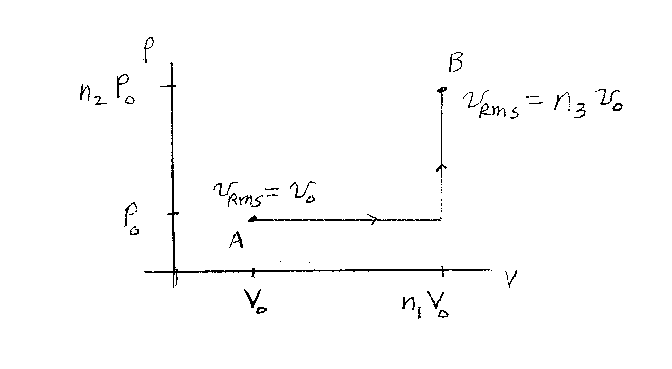Problem D19: An ideal gas starts out in the state A as shown in the figure below. State A has a volume of V0 and a pressure of P0. The RMS speed of the molecules in the state A is vrms = v0. The gas now undergoes a quasi-static process to the state B, which is first an isobaric and then an isometric process. The volume of state B is V = n1V0, and the pressure of state B is P = n2P0. If the RMS speed of the molecules in state B is vrms = n3 v0, what is n3? Note that n1, n2 and n3 are unitless.n1 = n2 = Input n3:

If you are currently in my class, you can record your grade by entering your name and student ID number (without the leading zeros) below and clicking on "record grade".
 First Name = Last Name = ID = Problem: